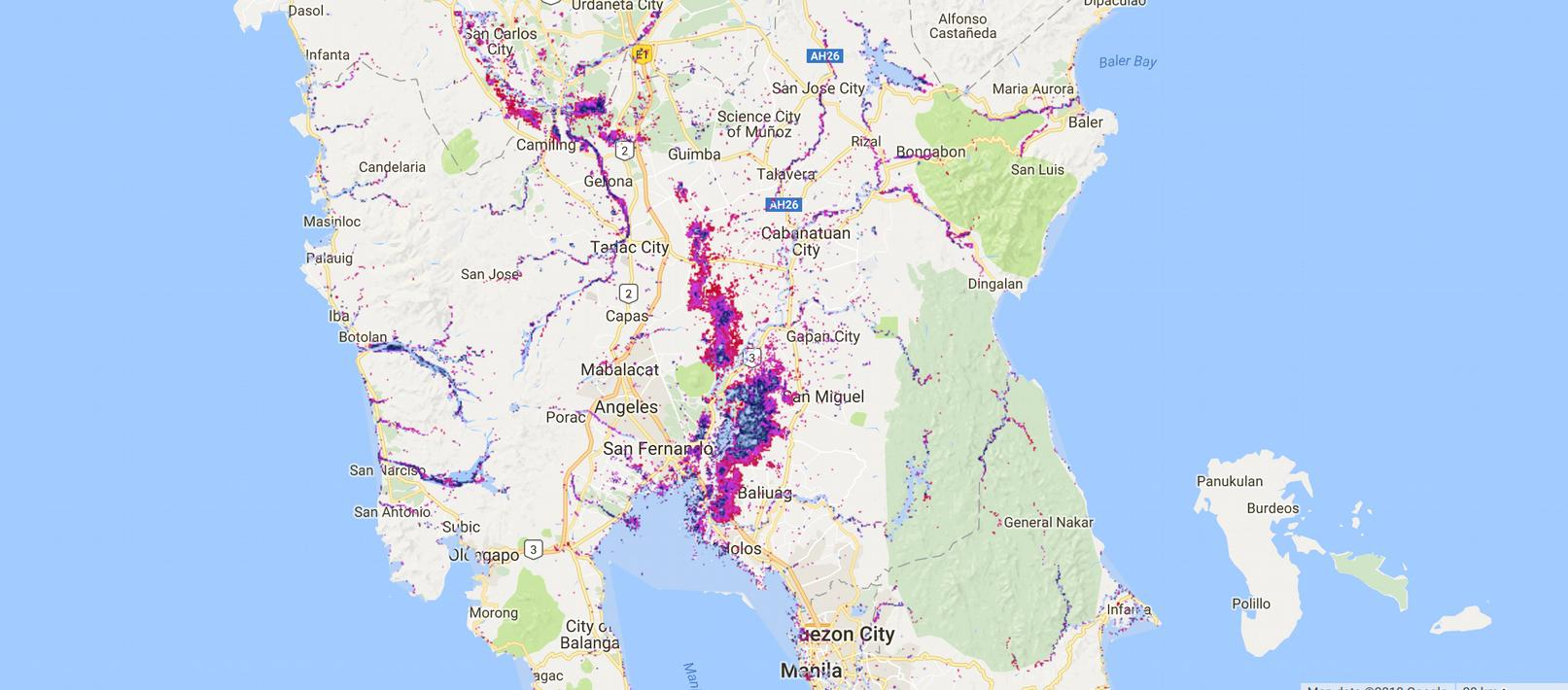# Philippines historical flood occurance

Calculate flood frequency from the JRC surface water

Step 1: import the JRC Monthly Water History v1.0 (1984-2015)

```var jrc = ee.ImageCollection("JRC/GSW1_0/MonthlyHistory");
```

Step 2: Set the geometry of the country

``` // Define country name
var country_names = ['Philippines'];
// import the country feasture collection
var countries = ee.FeatureCollection('ft:1tdSwUL7MVpOauSgRzqVTOwdfy17KDbw-1d9omPw');
// find the countries in the country list
var Country = countries.filter(ee.Filter.inList('Country', country_names)).geometry();
```

Step 3: Define the study period

```// Define study period
var startDate = ee.Date.fromYMD(1985, 1, 1);
var endDate = ee.Date.fromYMD(2016, 12, 31);
```

Step 4: filter the data for the study period

```// filter jrc data for country and period
var myjrc = jrc.filterBounds(Country).filterDate(startDate, endDate);
```

Step 5: Set all observations to 1 and add the layer as a new bands

```// Detect observations
var myjrc = myjrc.map(function(img){
// observation is img &gt; 0
var obs = img.gt(0);
});
```

Step 6: Set all observations with water to 1 and add the layer as a new bands

```// Detect all observations with water
var myjrc = myjrc.map(function(img){
// if water
var water = img.select('water').eq(2);
});
```

Step 7: calculate total number of observations and number of observations with water

```// calculate the total amount of observations
var totalObs = ee.Image(ee.ImageCollection(myjrc.select("obs")).sum().toFloat());
// calculate all observations with water
var totalWater = ee.Image(ee.ImageCollection(myjrc.select("onlywater")).sum().toFloat());
```

Step 8: Calculate the percentage of observations with water.

```// calculate the percentage of observations with water
var floodfreq = totalWater.divide(totalObs).multiply(100);
```

Step 9: Mask areas without water

```// maks areas that are not water
```

Step 10: visualize the result.

```var viz = {min:0, max:50, palette:['c10000,d742f4,001556,b7d2f7']};
1.Nitesh Shrestha says:
1.thisearthsite says: